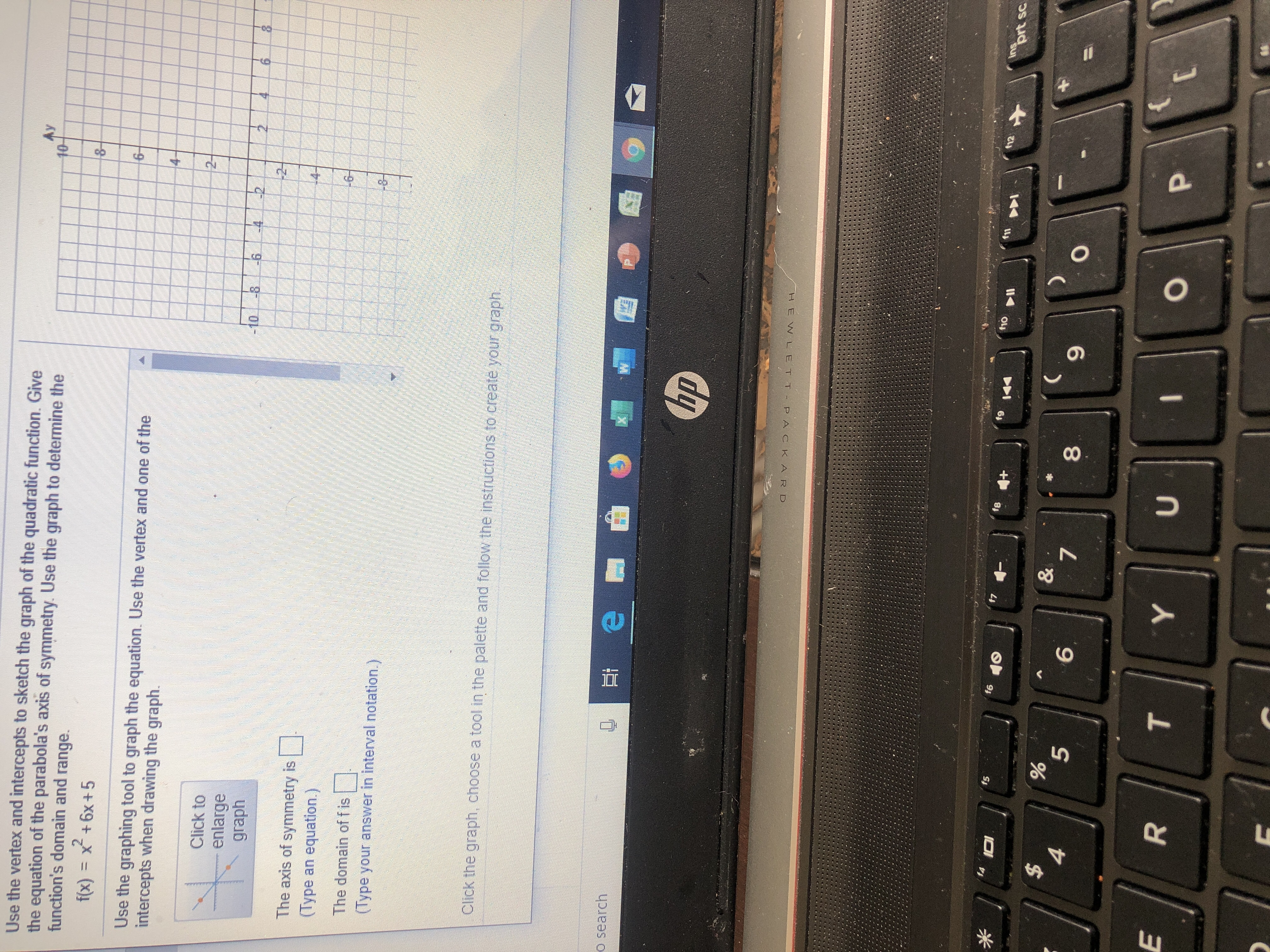Use the vertex and intercepts to sketch the graph of the quadratic function. Givethe equation of the parabola's axis of symmetry. Use the graph to determine thefunction's domain and range.Ay108-f(x) = x +6x+56Use the graphing tool to graph the equation. Use the vertex and one of theintercepts when drawing the graph.2Click toenlargegraph246 810-8-6.4The axis of symmetry is(Type an equation.)The domain of fis(lype your answer in interval notation.)Click the graph, choose a tool in the palette and follow the instructions to create your graphO searchhpHEW LET T P ACKARDinsprt sc12f9fef7f6f514+&7%5\$6PUEYTO00R

Questionhelp_outlineImage TranscriptioncloseUse the vertex and intercepts to sketch the graph of the quadratic function. Give the equation of the parabola's axis of symmetry. Use the graph to determine the function's domain and range. Ay 10 8- f(x) = x +6x+5 6 Use the graphing tool to graph the equation. Use the vertex and one of the intercepts when drawing the graph. 2 Click to enlarge graph 24 6 8 10-8-6.4 The axis of symmetry is (Type an equation.) The domain of fis (lype your answer in interval notation.) Click the graph, choose a tool in the palette and follow the instructions to create your graph O search hp HEW LET T P ACKARD ins prt sc 12 f9 fe f7 f6 f5 14 + & 7 % 5 \$ 6 P U E Y T O 00 R fullscreen
Step 1

In order to sketch the graph of quadratic function, convert the given quadratic function into standard form as follows.

Step 2

From the above standard form, observed that the axis of symmetry is x + 3 = 0 which can be written as x = -3. Substitute x = 0 in the given equation.

Step 3

Thus, the y-intercept of the given function is y = 5. Substitute f = 0 i...

Want to see the full answer?

See Solution

Want to see this answer and more?

Our solutions are written by experts, many with advanced degrees, and available 24/7

See Solution
Tagged in

Algebra